Courses

# Test: Basic Electrical Components

## 15 Questions MCQ Test Sensor & Industrial Instrumentation | Test: Basic Electrical Components

Description
This mock test of Test: Basic Electrical Components for GATE helps you for every GATE entrance exam. This contains 15 Multiple Choice Questions for GATE Test: Basic Electrical Components (mcq) to study with solutions a complete question bank. The solved questions answers in this Test: Basic Electrical Components quiz give you a good mix of easy questions and tough questions. GATE students definitely take this Test: Basic Electrical Components exercise for a better result in the exam. You can find other Test: Basic Electrical Components extra questions, long questions & short questions for GATE on EduRev as well by searching above.
QUESTION: 1

### What is the voltage across the capacitor in the given figure 35s after the input voltagesteps from 0V to 54V, if the value of the resistor is 47 kΩ and the capacitor is 1.5F?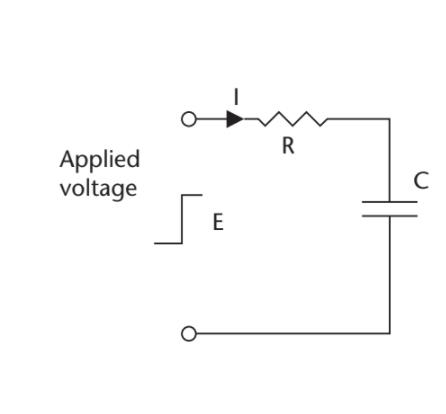Solution: Ec = 54(1 − e−35 × 1000000/1000 × 47 × 1000 × 1.5)V = 54 (1 − 1/e0.5)V

Ec = 54(1 − 0.606) = 21.3V

In an RC network when a step voltage is applied, the voltage across the capacitor is given by the equation

Ec = E{1− e-(t/RC)}

where Ec is the voltage across the capacitor at any instant of time, E is the source

voltage, t is the time (seconds) after the step is applied, R is in ohms and C is in farads.

QUESTION: 2

### What is the time constant for the circuit shown in the given figure, if the resistor has avalue of 220 kΩ, and the capacitor is 2.2 F?Solution: t = 2.2 × 10−6 × 220 × 103 sec = 484 × 10−3 sec = 0.484 sec

The time constant of the voltage in a capacitive circuit is defined as:

t = CR

where t is the time (seconds) it takes for the voltage to reach 63.2% of its final or

aiming voltage after the application of an input voltage step (charging or discharging).

QUESTION: 3

### The resistive elements in a strain gauge are each 5kΩ. A digital voltmeter with ranges of 10V, 1V, and 0.1V, and a resolution of 0.1% of FSD, is used to measure the output voltage. If R2 is the fixed element and R1 is the element measuring strain, what is the minimum change in R1 that can be detected? Assume a supply of 10V.

Solution: To measure the output voltage ≈ 5V, the 10V range is required, giving a resolution or sensitivity of 10 mV.

VR = 5.01V = 5,000 × 10/(R1 + 5,000)V

5.01(R1 + 5,000) = 50,000

R1 = (50,000/5.01) − 5,000 = 4,980Ω

Resolution = 5,000 − 4,980 = 20Ω

QUESTION: 4

Resistors R1 and R2 in the bridge circuit shown in the given figure are the strain gauge elements of 5kΩ each. Resistors R3 and R4 are fixed resistors, with values of 4.3 kW. A digital voltmeter with ranges of 10V, 1V, and 0.1V, and a resolution of 0.1% of FSD, is used to measure the output voltage. What is the minimum change in R1 that can be detected by the meter? Assume the supply E is 10V.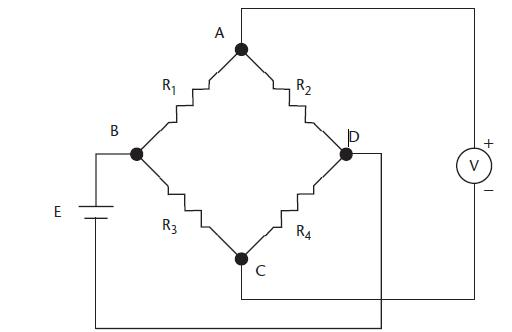Solution: The voltage at point C will be 5.0V, because R3 = R4, and the voltage at C equals one-half of the supply voltage. The voltmeter can use the 0.1V range, because the offset is 0V, giving a resolution of 0.1 mV. The voltage that can be sensed at A is given by

EAD = 10 × R2/(R1 + R2) = 5.00 + 0.0001V

R1 = (50,000/50,001) − 5,000Ω

R1 = −49,999

Resolution = 1Ω

QUESTION: 5

If the bridge circuit in the given figure is balanced, with R1 = 15 kΩ, R2 = 27 kΩ, R3= 18 kΩ, and C1 = 220 pF, what are the values of R4 and C2?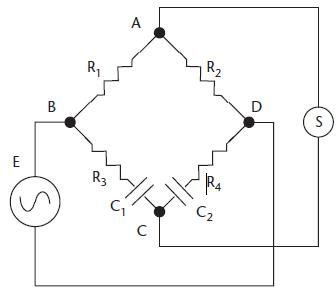Solution: R2R3 = R1R4

R4 = 27 × 18/15 = 32.4 kΩ

C2R2 = C1R1

C2 = 220 × 15/27 = 122 pF

QUESTION: 6

In the potentiometer circuit shown in the figure, the expression for Vx is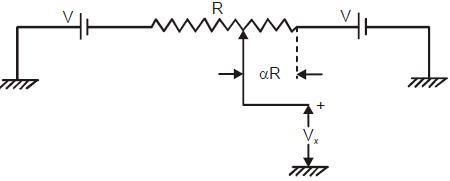Solution:

Using KVL,

Vx + (αR) 2V/R - V = 0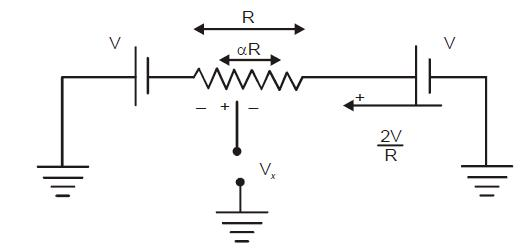QUESTION: 7

The response of a first-order measurement system to a unit step input is 1 – e– 0.5t, where t is in seconds. A ramp of 0.1 units per second is given as the input to this system. The error in the measured value after transients have died down is

Solution: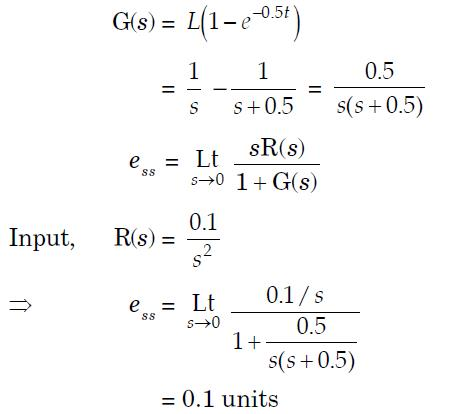QUESTION: 8

A metal wire has a uniform cross-section A, length, and resistance R between its two endpoints. It is uniformly stretched so that its length becomes l. The new resistance is

Solution:

Volume is constant

lA = l’A’

lA = α l A’

A’ = A/α

R’ = ρl'/A' = ρ αlα/A = α2 R

QUESTION: 9

A variable air gap type capacitor consists of two parallel plates: a fixed plate and a moving plate at a distance x. If a potential V is applied across the two plates, then the force of attraction between the plates is related to x as

Solution:

E = ½ CV2. = ½  εA/x V2

F = -δEX = ½  εA/xx V2 which is inversely proportional to x2

QUESTION: 10

The expression for the capacitance (C in pF) of a parallel plate capacitor is given by:

C = 6.94 × 10– 3 (d2/S). The diameter (d) of each plate is 20 mm and the spacing between the plates

(S) is 0.25 mm. The displacement sensitivity of the capacitor is approximate:

Solution:

C = 6.94 x 10- 3 (d2/S) PF

Displacement sensitivity = dC/dS = -6.94 x 10 - 3 x 20 2 / (0.25)2 = - 44.4 pF/mm

QUESTION: 11

Which of the following can be measured using a Wheatstone bridge?

Solution: Wheatstone bridge cannot be limited to measurement of a single quantity only, it can be used to measure resistance, capacitance, impedance, inductance etc. with some modifications.
QUESTION: 12

Accuracy in a bridge measurement depends on

Solution: Both sensitivity of indicator and applied voltage affects the accuracy in bridge measurement.
QUESTION: 13

In a Wheatstone bridge, which of the following is used as a null detector?

Solution: Null detector is used to indicate zero current flow, and the device used is a galvanometer.
QUESTION: 14

Which of the following statements is correct for impedance?

Solution: Impedance of pure resistance is purely real and for an inductor purely imaginary, where the inductor is a reactive component.
QUESTION: 15

What is the purpose of Owens Bridge?

Solution: Owens Bridge is an AC bridge that is used to measure reactance.